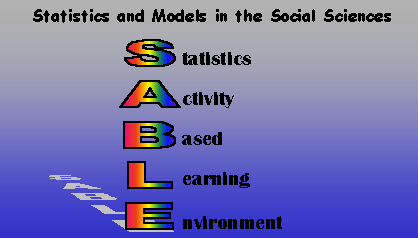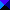Welcome to SABLE, Virginia Tech's Statistics Activity-Based Learning Environment for integrating statistics education into the Social Sciences curricula. The Learning Environment consists of interactive tutorials in which the student completes tasks that lead to an understanding of statistics principles and their application to social sciences data. Each tutorial employs visualizations of data and relationships and also allows the student to download and interact with data sets collected in the General Social Survey and others. The Learning Environment experience culminates in the student's use of the visualizations to analyze and draw conclusions from real data.

About the SABLE project at Virginia Tech, supported by FIPSE

All files, sofware, and tutorials that make up SABLE are Copyright (c) 1997 1998 1999 Virginia Tech. You may use these programs under the conditions of the SABLE General License, which incorporates the GNU GENERAL PUBLIC LICENSE.

 CONTENTS

Click thefor an overview of topics covered.

Introduction

Foundations
Making Decisions
Examining More Than One Variable

 SUMMARY OF TUTORIALS

(*) Denotes an Interactive Exercise

How to Use SABLE

Instructions for Using the SABLE Tutorials

Measurement Scales in Social Sciences Reseach

Introduction
Nominal Data
Ordinal Data
Interval Data
Ratio Data

Measures of Central Tendency: Mean, Median, and Mode

Introduction
The Frequency Histogram
Central Tendency
Ways to Measure Central Tendency *
Mean
Median
Mode
Which Measure Should You Use? *
Skewness ***

Sampling

Introduction
How can samples reveal information about a population? *
Sample characteristics
Randomness & representativeness *
Sampling Protocols
Central Limit Theorem
Stratification *

Measures of Dispersion: Departures from Central Tendency

Introduction
Range *
Percentiles & Related Characteristics **
Variance & Standard Deviation
Comparison of Range, Quartiles, and Standard Deviations
Standard Distribution Scores, or "Z-scores" **

Measures of Dispersion: Departures from Normal Distribution

The Normal Distribution
Non-Normal Distributions
Hypothesis Testing
Sampling and Testing Hypotheses **
Type I Errors
Type II Errors
Sampling Distributions
Standard Error
Confidence Interval
Critical Value
Statistical Significance
Null Hypothesis
Alternative Hypothesis
Non-directional Alternative Hypothesis
The Researcher's Dilemma
Fail to Reject
T-Distributions
Estimating Standard Error
Relationship Between the T-Distribution and the Normal Distribution
Single Sample T-Test
Independent Sample T-Test
Paired T-Test
Using the T-Statistic to Compare Regression Coefficients
Analysis of Variance
Sources of Variation *
Total Variation
Variation Within Groups
Variation Between Groups
Analysis of Variance Table *
Ratios Between SSs *
The F-ratio and the F-distribution *
One-Way Analysis of Variance (recap)
Two-way Analysis of Variance *
Recap and Summary
Cross Tabulations
Correlation & Covariance
Correlation *
Units of Analysis in Frequency Distributions *
Correlation and Standardized Distribution Scores *
Correlation vs. Causation
Correlation & Coefficient of Determination *
Covariance *
Linear Regression
Relating Variables **
Predicting Values for Variables *
Assumptions of Regression Analysis *
Multiple Regression